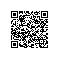# <转>C++ explicit关键字详解

class CxString  // 没有使用explicit关键字的类声明, 即默认为隐式声明
{
public:
char *_pstr;
int _size;
CxString(int size)
{
_size = size;                // string的预设大小
_pstr = malloc(size + 1);    // 分配string的内存
memset(_pstr, 0, size + 1);
}
CxString(const char *p)
{
int size = strlen(p);
_pstr = malloc(size + 1);    // 分配string的内存
strcpy(_pstr, p);            // 复制字符串
_size = strlen(_pstr);
}
// 析构函数这里不讨论, 省略...
};

// 下面是调用:

CxString string1(24);     // 这样是OK的, 为CxString预分配24字节的大小的内存
CxString string2 = 10;    // 这样是OK的, 为CxString预分配10字节的大小的内存
CxString string3;         // 这样是不行的, 因为没有默认构造函数, 错误为: “CxString”: 没有合适的默认构造函数可用
CxString string4("aaaa"); // 这样是OK的
CxString string5 = "bbb"; // 这样也是OK的, 调用的是CxString(const char *p)
CxString string6 = 'c';   // 这样也是OK的, 其实调用的是CxString(int size), 且size等于'c'的ascii码
string1 = 2;              // 这样也是OK的, 为CxString预分配2字节的大小的内存
string2 = 3;              // 这样也是OK的, 为CxString预分配3字节的大小的内存
string3 = string1;        // 这样也是OK的, 至少编译是没问题的, 但是如果析构函数里用free释放_pstr内存指针的时候可能会报错, 完整的代码必须重载运算符"=", 并在其中处理内存释放  

CxString string2(10);

CxString temp(10);
CxString string2 = temp;


但是, 上面的代码中的_size代表的是字符串内存分配的大小, 那么调用的第二句 "CxString string2 = 10;" 和第六句 "CxString string6 = 'c';" 就显得不伦不类, 而且容易让人疑惑. 有什么办法阻止这种用法呢? 答案就是使用explicit关键字. 我们把上面的代码修改一下, 如下:

class CxString  // 使用关键字explicit的类声明, 显示转换
{
public:
char *_pstr;
int _size;
explicit CxString(int size)
{
_size = size;
// 代码同上, 省略...
}
CxString(const char *p)
{
// 代码同上, 省略...
}
};

// 下面是调用:

CxString string1(24);     // 这样是OK的
CxString string2 = 10;    // 这样是不行的, 因为explicit关键字取消了隐式转换
CxString string3;         // 这样是不行的, 因为没有默认构造函数
CxString string4("aaaa"); // 这样是OK的
CxString string5 = "bbb"; // 这样也是OK的, 调用的是CxString(const char *p)
CxString string6 = 'c';   // 这样是不行的, 其实调用的是CxString(int size), 且size等于'c'的ascii码, 但explicit关键字取消了隐式转换
string1 = 2;              // 这样也是不行的, 因为取消了隐式转换
string2 = 3;              // 这样也是不行的, 因为取消了隐式转换
string3 = string1;        // 这样也是不行的, 因为取消了隐式转换, 除非类实现操作符"="的重载


explicit关键字的作用就是防止类构造函数的隐式自动转换.

class CxString  // explicit关键字在类构造函数参数大于或等于两个时无效
{
public:
char *_pstr;
int _age;
int _size;
explicit CxString(int age, int size)
{
_age = age;
_size = size;
// 代码同上, 省略...
}
CxString(const char *p)
{
// 代码同上, 省略...
}
};


但是, 也有一个例外, 就是当除了第一个参数以外的其他参数都有默认值的时候, explicit关键字依然有效, 此时, 当调用构造函数时只传入一个参数, 等效于只有一个参数的类构造函数, 例子如下:

class CxString  // 使用关键字explicit声明
{
public:
int _age;
int _size;
explicit CxString(int age, int size = 0)
{
_age = age;
_size = size;
// 代码同上, 省略...
}
CxString(const char *p)
{
// 代码同上, 省略...
}
};

// 下面是调用:

CxString string1(24);     // 这样是OK的
CxString string2 = 10;    // 这样是不行的, 因为explicit关键字取消了隐式转换
CxString string3;         // 这样是不行的, 因为没有默认构造函数
string1 = 2;              // 这样也是不行的, 因为取消了隐式转换
string2 = 3;              // 这样也是不行的, 因为取消了隐式转换
string3 = string1;        // 这样也是不行的, 因为取消了隐式转换, 除非类实现操作符"="的重载


以上即为C++ explicit关键字的详细介绍.使用钉钉扫一扫加入圈子
+ 订阅